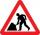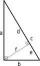Diagonal

Calculate the length of the diagonal of the rectangle ABCD with sides a = 8 cm, b = 7 cm.

Result

u =  10.6 cm

Solution:Leave us a comment of example and its solution (i.e. if it is still somewhat unclear...):

Showing 1 comment:Math studentTo solve this example are needed these knowledge from mathematics:

Pythagorean theorem is the base for the right triangle calculator. See also our trigonometric triangle calculator.

Next similar examples:

1. Diagonal - simpleCalculate the length of the diagonal of a rectangle with dimensions 5 cm and 12 cm.
2. MedianIn the right triangle are sides a=41 dm b=42 dm. Calculate the length of the medians tc to the hypotenuse.
3. Tv screenThe size of a tv screen is given by the length of its diagonal. If the dimension of a tv screen is 16 inches by 14 inches, what is the size of the tv screen?
4. SatinSanusha buys a piece of satin 2.4 m wide. The diagonal length of the fabric is 4m. What is the length of the piece of satin?
5. StairwayStairway has 20 steps. Each step has a length of 22 cm and a height of 15 cm. Calculate the length of the handrail of staircases if on the top and bottom exceeds 10 cm.
6. Chord circleThe circle to the (S, r = 8 cm) are different points A, B connected segment /AB/ = 12 cm. AB mark the middle of S'. Calculate |SS'|. Make the sketch.
7. Four ropesTV transmitter is anchored at a height of 44 meters by four ropes. Each rope is attached at a distance of 55 meters from the heel of the TV transmitter. Calculate how many meters of rope were used in the construction of the transmitter. At each attachment.The ladder has a length 3.5 meters. He is leaning against the wall so that his bottom end is 2 meters away from the wall. Determine the height of the ladder.
9. BaseCompute base of an isosceles triangle, with the arm a=20 cm and a height above the base h=10 cm.The double ladder shoulders should be 3 meters long. What height will the upper top of the ladder reach if the lower ends are 1.8 meters apart?
11. WindbreakA tree at a height of 3 meters broke in the windbreak. Its peak fell 4.5 m from the tree. How tall was the tree?8.3 meters long ladder is leaning against the wall of the well, and its lower end is 1.2 meters from this wall. How high from the bottom of a well is the top edge of the ladder?The tree was 35 meters high. The tree broke at a height of 10 m above the ground. Top but does not fall off it refuted on the ground. How far from the base of the tree lay its peak?Oil drilling rig is 23 meters height and fix the ropes which ends are 7 meters away from the foot of the tower. How long are these ropes?The double ladder is 8.5m long. It is built so that its lower ends are 3.5 meters apart. How high does the upper end of the ladder reach?Ladder 6.4 meters long is positioned in the well such that its lower end is distanced from the wall of the well 1.2 m. The upper part of the ladder is supported on the upper edge of the well. How high is the well?It is true that the middle traverse bisects the triangle?